• fifo lru
2021-11-11 21:14:14

注：笔记是对fengsigaoju大佬的实现页面置换算法一文（(35条消息) JAVA实现页面置换算法（OPT,FIFO,LRU）_fengsigaoju的博客-CSDN博客_页面置换算法代码java）的学习，侵删。

初学一个月JAVA，如有不足请指出。

先上代码：

import java.io.BufferedInputStream;
import java.util.*;

public class Main {
private int n;//内储页框
private int m;//访问次数
private int F;//没能直接找到的次数,(F/m)为缺页率
private List<Integer>list=null;//访问地址走向
private Map<Integer,Integer>map=null;
public Main(){
F=0;
map=new HashMap<Integer,Integer>();//存储每一个内储页框所存的内容
Scanner cin=new Scanner(new BufferedInputStream(System.in));
System.out.println("请输入用户访问页地址走向");
list=new ArrayList<Integer>();
String s=cin.nextLine();
String []s1=s.split(" ");
m=s1.length;
for (int i=0;i<m;i++)
System.out.println("请输入内储叶框数量");
n=cin.nextInt();
switch(cin.nextInt()){
case 1:OPT();break;
case 2:FIFO();break;
case 3:LRU();break;
case 4:LFU();break;
}
cin.close();
}
System.out.println("**** 选择最佳置换算法请按1 **********");
System.out.println("**** 选择先进先出置换算法请按2 *******");
System.out.println("**** 选择最近最远未使用置换算法请按3 ***");
System.out.println("**** 使用lfr请按4 ******************");
}
public void OPT(){//最佳置换算法
int j;
for (int i=0;i<m;i++)
{
int k=list.get(i);//待处理元素
//System.out.print(map);
if (!map.containsValue(k)){
F++;//不能直接找到次数加1
if (map.size()<n){//如果没有装满
int temp=map.size();
map.put(temp, k);
}
else{//如果装满了
int index=0;//把哪个位置的淘汰出去
int min=0;//初始最长长度
for (int t=0;t<n;t++)
{
for (j=i+1;j<m;j++){//看后面哪一个出现的最晚
if (list.get(j)==map.get(t)){//第一次找到
if (j-i>min){
index=t;//更新值
min=j-i;
}
break;
}
}
if (j==m){//如果到最后
index=t;
min=j-i;
}
}
map.remove(index);
map.put(index,k);//修改表内元素
}
}
}
System.out.println("误码率为:"+F*1.0/m);
}
public void FIFO(){//先进先出置换算法
for (int i=0;i<m;i++)
{
int k=list.get(i);//待处理元素
if (!map.containsValue(k)){
F++;//不能直接找到次数加1
if (map.size()<n){//如果没有装满
int temp=map.size();
map.put(temp, k);
q.offer(temp);
}
else
{
int temp=q.poll();//排除的元素位置
map.remove(temp);
map.put(temp,k);
q.offer(temp);

}
}
}
System.out.println("误码率为:"+F*1.0/m);
}
public void LRU(){//最近最远未使用置换算法
int start=0;
for (int i=0;i<m;i++)
{
int k=list.get(i);//待处理元素
if (!map.containsKey(k)){
F++;//不能直接找到次数加1
if (map.size()<n){//如果没有装满
int temp=map.size();
map.put(k,temp);
}
else
{
int c=map.get(temp);//位置
map.remove(temp);
map.put(k,c);
}
}
else//如果包含这个值，把这个值拿走并在后面压入
{
}
}
System.out.println("误码率为:"+F*1.0/m);
}
public void LFU() {
for (int i=0;i<m;i++)
{
int k=list.get(i);//待处理元素
if (!map.containsValue(k)){
F++;
if (map.size()<n){
int temp=map.size();
map.put(temp, k);
}
else{
int index=0;
int min=0;
int num=0;
for (int t=0;t<n;t++)
{
for (int j=i-1;j>0;j--){
if (list.get(j)==map.get(t)){
num++;
}
if(min<num){
min=num;
index=t;
}
}
}
map.remove(index);
map.put(index,k);
}
}
}
System.out.println("误码率为:"+F*1.0/m);
}
public static  void main(String args[]){
Main p=new Main();
}
}

不得不说，就我而言的话，我认为这段代码还是写的很棒滴。

第四种方法LFU是我自己加的，写的不是特别好，但是满足了教科书所要求的四种方法。

至于CLOCK算法，有空了再更。

接下来是对这段代码的理解：

一、使用到的代码：

1.第一行的import BufferedInputStream对于初学者来说有点深入了，当此行去掉，将原有的

Scanner cin=new Scanner(new BufferedInputStream(System.in));

改为

Scanner cin=new Scanner(System.in);

也可正常运行；

2.List

List <integer> list=new ArrayList<Integer>();

3.HashMap

哈希表是一个非常实用的类型，主要特征是有key-value键值对，单从这一点容易让人联系到Python中的字典，但功能远比其丰富。

很多的内置函数都是可以从字面判断出来的：

containsValue(k)在表中查找是否有k的value。

size()返回键值对的数量。

put() 添加或者修改键值对。

remove()移出键值对，返回值为删除的值。

get()返回键所对应的值。

4.Queue

q.remove:和pop一样是删除头元素，在空队列时，remove报错，pop返回null。

链表，算是非常常见的类型吧，这里就不多赘述了。

二、阅读代码遇到的问题

1、阅读LRU（）的时候那个temp指针把我弄晕了。。。后来上编译器才发现这两个if里头的temp压根不一样。。。可见编译器的重要性了

三、总结

总的来说还是比较基础的代码，学校实验真是难的一批，写写这方面的笔记找找灵感。本来想写长一点的结果女朋友叫我帮她写Python去。。

这是学了一个月的菜鸡的笔记，有错误还请大佬指正。

一天后更：

果然写笔记是有帮助的，今天实验一下子就打通思路了。

还有就是对前文的一些错误进行纠错。java 开发语言 后端 apache
更多相关内容
• c++实现操作系统请求调页功能 分别有FIFO LRU 和OPT 算法
• 第 PAGE 8 页 共 NUMPAGES13 页 操作系统原理 上机作业报告 作业 页 面 淘 汰 算 法 作业编号 6 题目 页面淘汰/置换算法 作业要求 题目要求通过模拟...针对一个页框根据实验数据以OPT算法为参考研究FIFO页面淘汰算法LRU
• 存储管理详细实验报告和cpp文件，含FIFOLRU的比较，实验报告都是我一个一个字敲进去的。
• 国内常见的课程项目。本实验的目的是通过请求页式存储管理中页面置换算法模拟设计，了解虚拟存储技术的特点，掌握请求页式存储管理的页面置换算法。c++
• 三种缓存机制：FIFO / LRU / LFU

# 目录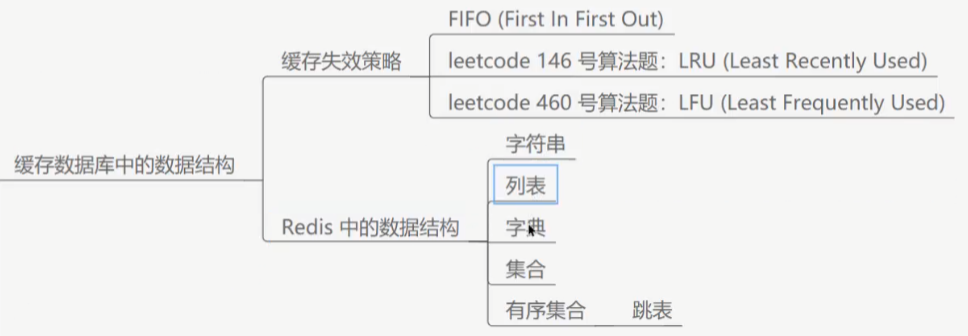## 缓存的概念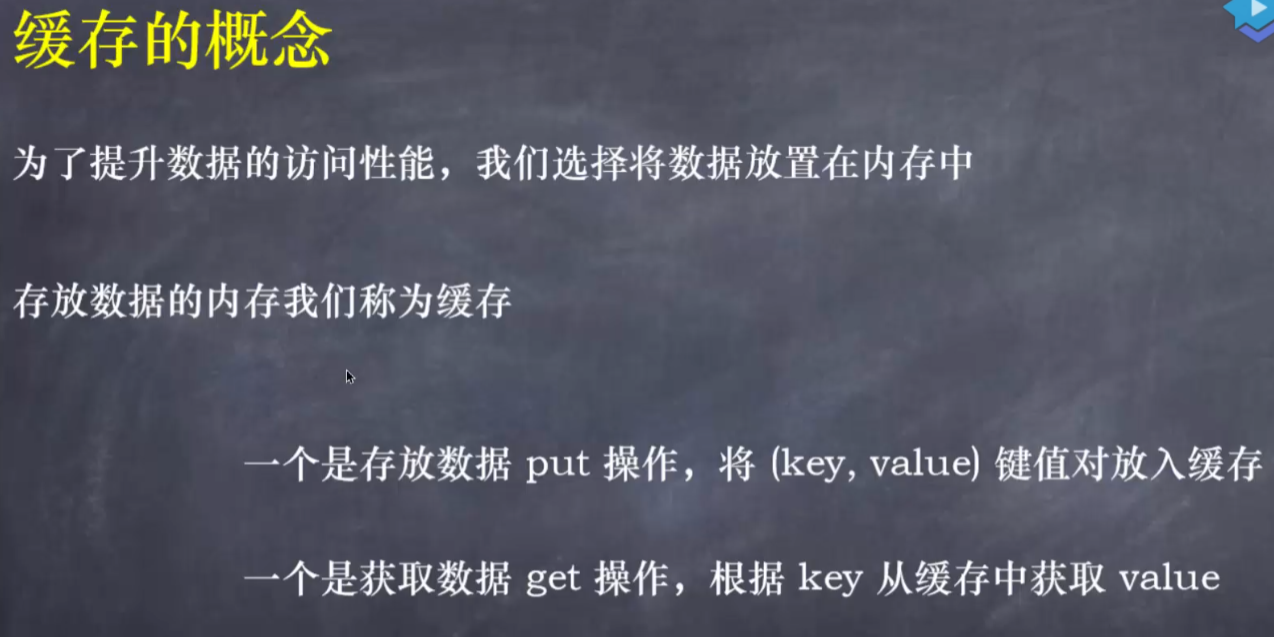## 定义cache接口

/**
* @author zzyuan
* @date 2021/11/3 - 21:07
*/
//缓存接口
public interface Cache<K,V> {
//取
V get(K key);
//存
void put(K key , V value);
}



## 无限容量的缓存数据结构代码

使用内置的HashMap

/**
* @author zzyuan
* @date 2021/11/3 - 21:09
*/
public class LocalCache<K,V> implements Cache<K,V> {

private Map<K,V> cache;

public LocalCache() {
cache = new HashMap<>();
}

@Override
public V get(K key) {
return cache.get(key);
}

@Override
public void put(K key, V value) {
cache.put(key,value);
}
}



缺点：
无限容量的缓存我们底层可以使用HashMap
但是实际上缓存容量是有限的

## 缓存的淘汰机制(FIFO)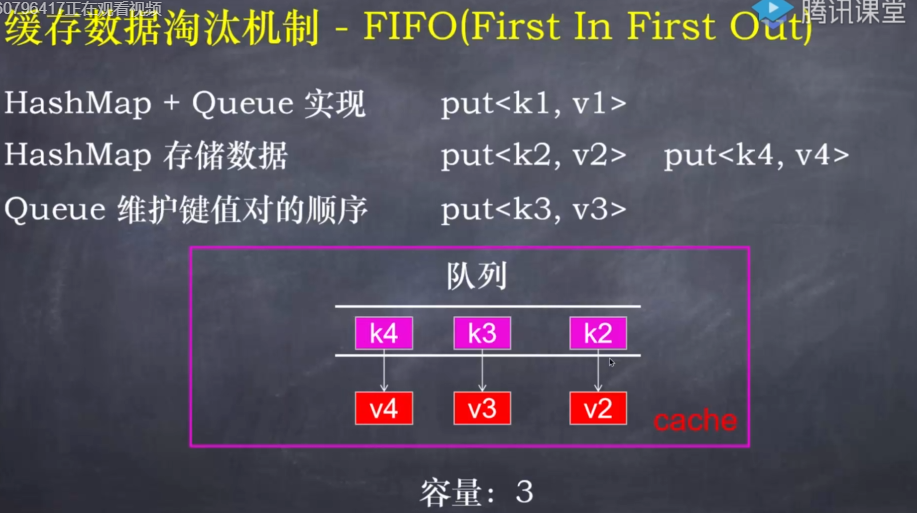HashMap + Queue实现

/**
* @author zzyuan
* @date 2021/11/3 - 21:09
*/
public class FIFOCache<K,V> implements Cache<K,V> {

private Map<K,V> cache;
private Queue<K> queue;
private int capacity;//缓存最大的容量

public FIFOCache(int capacity) {
this.cache = new HashMap<>(capacity);
//循环队列
this.queue = new ArrayDeque<>(capacity);
this.capacity = capacity;
}

//取元素：o(1)
@Override
public V get(K key) {
return cache.get(key);
}

@Override
public void put(K key, V value) {
//先判断要存入的值是否已经存在
V oldValue = cache.get(key);

//不存在该缓存
if(oldValue == null){
//我们要检测一下缓存容量是否已经满了
//如果缓存满了：FIFO机制删除缓存
if(cache.size() == capacity){
//删除队头元素
K oldKey = queue.poll();
//把要淘汰的元素在缓存中移除
cache.remove(oldKey);
}
//然后再新 key 存入到队尾
queue.offer(key);
}
//最后存入到缓存中
cache.put(key,value);
}

//测试样例
public static void main(String[] args) {
FIFOCache<Integer,Integer> cache = new FIFOCache<>(3);
cache.put(1,1);
cache.put(2,2);
cache.put(3,3);
cache.put(4,4);
System.out.println(cache.get(3));
cache.put(4,5);
System.out.println(cache.get(4));
cache.put(5,6);
System.out.println(cache.get(2));
}
}



## 缓存的淘汰机制(LRU) (重点!!)

双向链表的表头是最近使用的数据
双向链表的表尾是最久被被使用的数据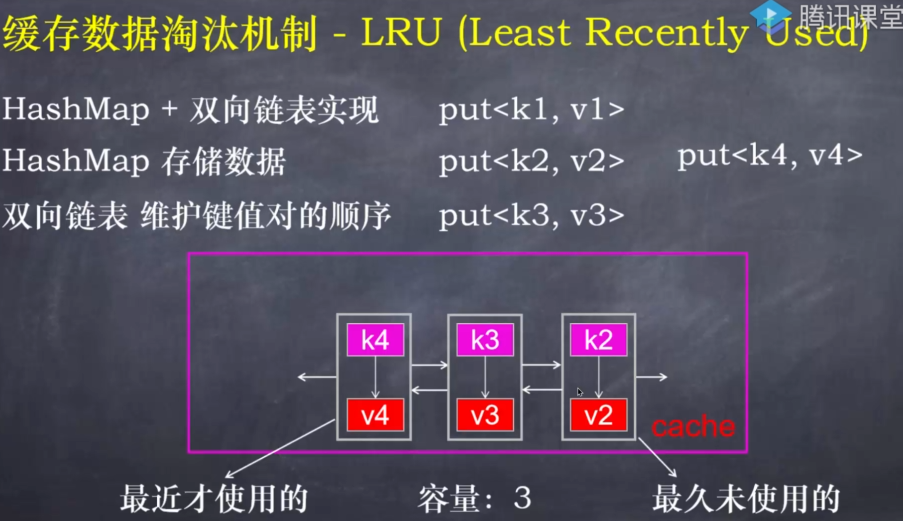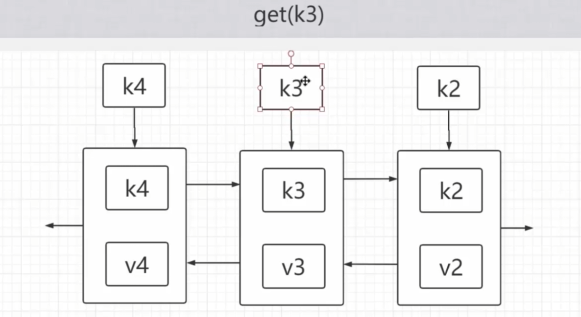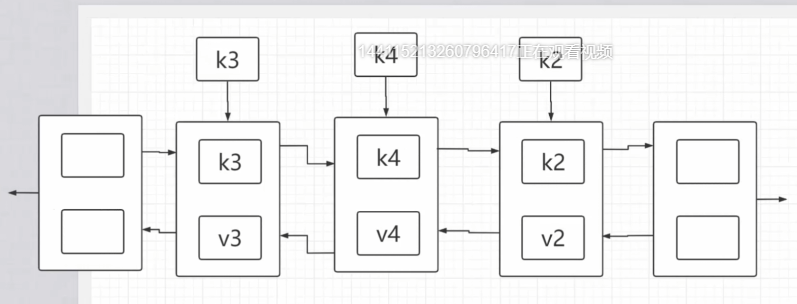/**
* @author zzyuan
* @date 2021/11/3 - 21:09
*/

/**
* 双向链表的表头是最近使用的数据
* 双向链表的表尾是最久被被使用的数据
*
* @param <K>
* @param <V>
*/
public class LRUCache<K,V> implements Cache<K,V> {

private class Node{
K key;
V value;
Node next;
Node prev;
}

private Map<K,Node> cache;//根据key快速拿到 Node
private int capacity;
private Node tail;//虚拟尾结点

public LRUCache(int capacity) {
tail = new Node();

cache = new HashMap<>(capacity);
this.capacity = capacity;
}

@Override
public V get(K key) {
Node node = cache.get(key);
if(node == null) return null;
//将查询到的结点移动到链表的表头
return node.value;
}

//1.删除结点Node
removeNode(node);

//2.将结点node添加到表头
}

//删除结点Node
private void removeNode(Node node) {
Node preNode = node.prev;
Node nextNode = node.next;

preNode.next = nextNode;
nextNode.prev = preNode;

node.prev = null;
node.next = null;
}

// 将一个节点添加到头节点

}

@Override
public void put(K key, V value) {
Node node = cache.get(key);
//Node 不存在
if(node == null){
//1.判断缓存容量大小
//  1.1 容量如果超了,删除链表的尾结点
if(cache.size() == capacity){
Node delNode = removeTailNode();
cache.remove(delNode.key);
}
//2.创建结点
node = new Node();
node.key = key;
node.value = value;
//3.维护链表和缓存
cache.put(key,node);
}else{//Node存在
node.value = value;
// 有的话，则将节点放到头结点
}
}

//删除尾结点返回Node为了使得map中取key删除结点值
private Node removeTailNode() {
Node delNode = tail.prev;
removeNode(delNode);
return delNode;
}

//测试用例
public static void main(String[] args) {
LRUCache<Integer,Integer> cache = new LRUCache<>(3);
cache.put(1,1);
cache.put(2,2);
cache.put(3,3);
cache.put(4,4);
System.out.println(cache.get(3));
cache.put(2,5);
cache.put(5,6);
System.out.println(cache.get(4));
}

}



java内置的数据结构支持LRU缓存机制

public class LinkedHashMapTest {
public static void main(String[] args) {
// 1. LinkedHashMap 会维护 put 的键值对的相对顺序
// 2. 维护最近使用的键值对，放在表头，表尾的键值对就是最久未使用的
Map<Integer, Integer> map = new LinkedHashMap<>(3, 0.75F, true);

map.put(5, 5);
map.put(2, 2);
map.put(9, 9);

for (Map.Entry<Integer, Integer> entry : map.entrySet()) {
System.out.println(entry.getKey() + " " + entry.getValue());
}

System.out.println("--------------------------------------");

map.put(2, 10);
map.get(5);
for (Map.Entry<Integer, Integer> entry : map.entrySet()) {
System.out.println(entry.getKey() + " " + entry.getValue());
}
}
}

package com.douma.practical.cache;

import java.util.Map;

public class LRUCache1<K, V> extends LinkedHashMap<K, V> {
private int capacity;

public LRUCache1(int capacity, int initialCapacity, float loadFactor) {
this.capacity = capacity;
}

@Override
protected boolean removeEldestEntry(Map.Entry<K, V> eldest) {
if (size() > capacity) {
return true;
}
return false;
}

public static void main(String[] args) {
LRUCache1<Integer, Integer> cache =
new LRUCache1<>(3, 3, 0.75F);
cache.put(1, 1);
cache.put(2, 2);
cache.put(3, 3);
cache.put(4, 4);
System.out.println(cache.get(3));
cache.put(2, 5);
cache.put(5, 6);
System.out.println(cache.get(4));
}
}

展开全文缓存
• 实验四 页式虚拟存储管理中地址转换和页式中断 FIFO LRU OPT
• 共四种：FIFO\LRU\LFU\OPT 。在VC环境下运行完全成功。 存储管理的主要功能之一是合理地分配空间。请求页式管理是一种常用的虚拟存储管理技术。本实验目的是通过请求页式管理中页面置换算法模拟设计，了解虚拟存储...
• FIFO、OPT、LRU页面置换算法实验代码和截图操作系统
• 操 作 系 统 实 验 报 告 页面置换算法模拟 OFTFIFO 和 LRU 算法 班级2013 级软件工程 1 班 学号X X X 姓名萧氏一郎 开始载入序列 开始 载入序列号从第 0 个得到页号 将页号放入物理地址中编号 s 根据选择的置换算法...
• 先进先出的算法(FIFO) 最近最久未使用算法(LRU) 最不经常使用算法(LFU) 最近未使用算法(NUR) 命中率=1-页面失效次数/页地址流长度 首先用 srand( )和 rand( )函数定义和产生指令序列，然后将指令序列变换成相应的页...

设计一个虚拟存储区和内存工作区，并使用下述算法计算访问命中率.

1. 最佳淘汰算法(OPT)
2. 先进先出的算法(FIFO)
3. 最近最久未使用算法(LRU)
4. 最不经常使用算法(LFU)
5. 最近未使用算法(NUR)
命中率=1-页面失效次数/页地址流长度
首先用 srand( )和 rand( )函数定义和产生指令序列，然后将指令序列变换成相应的页地址流，并针对不同的算
法计算出相应的命中率。
通过随机数产生一个指令序列，共 320 条指令.指令的地址按下述原则生成:
1. 50%的指令是顺序执行的
2. 25%的指令是均匀分布在前地址部分
3. 25%的指令是均匀分布在后地址部分
具体的实现方法是:
4. 在[0，319]的指令地址之间随机选取一起点 m
5. 顺序执行一条指令，即执行地址为 m+1 的指令
6. 在前地址[0，m+1]中随机选取一条指令并执行，该指令的地址为 m
7. 顺序执行一条指令，其地址为 m+1
8. 在后地址[m+2，319]中随机选取一条指令并执行
9. 重复步骤 1-5，直到 320 次指令
将指令序列变换为页地址流
设:：页面大小为 1K；用户内存容量 4 页到 32 页；用户虚存容量为 32K。
在用户虚存中，按每 K 存放 10 条指令排列虚存地址，即 320 条指令在虚存中的存放方式为：第 0 条-第 9 条
指令为第 0 页(对应虚存地址为[0，9])，第 10 条-第 19 条指令为第 1 页(对应虚存地址为[10，19])，……，第
310 条-第 319 条指令为第 31 页(对应虚存地址为[310，319])。
按以上方式，用户指令可组成 32 页.
#include<stdlib.h>
#include <unistd.h>
#include <stdio.h>
#define TRUE 1
#define FALSE 0
#define INVALID -1
#define NULL 0
#define total_instruction 320 /*指令流长*/
#define total_vp 32 /*虚页长*/
#define clear_period 50 /*清 0 周期*/

typedef struct /*页面结构*/
{
int pn,pfn,counter,time;
}pl_type;

pl_type pl[total_vp]; /*页面结构数组*/

struct pfc_struct{ /*页面控制结构*/
int pn,pfn;
struct pfc_struct *next;
};

typedef struct pfc_struct pfc_type;
int diseffect, a[total_instruction];
int page[total_instruction], offset[total_instruction];
int initialize(int total_pf);
int FIFO(int total_pf);
int LRU(int total_pf);
int LFU(int total_pf);
int NUR(int total_pf);
int OPT(int total_pf);
int main( )
{
int s,i,j;
srand(10*getpid()); /*由于每次运行时进程号不同,故可用来作为初始化随机数队列的种子*/
s=(float)319*rand( )/32767/32767/2+1; //
for(i=0;i<total_instruction;i++)
{
if(s<0||s>319)
{
printf("When i==%d,Error,s==%d\n",i,s);
exit(0);
}
a[i]=s; /*任选一指令访问点 m*/
a[i+1]=a[i]+1; /*顺序执行一条指令*/
a[i+2]=(float)a[i]*rand( )/32767/32767/2; /*执行前地址指令 m */
a[i+3]=a[i+2]+1; /*顺序执行一条指令*/
s=(float)(318-a[i+2])*rand( )/32767/32767/2+a[i+2]+2;
if((a[i+2]>318)||(s>319))
printf("a[%d+2],a number which is :%d and s==%d\n",i,a[i+2],s);
}
for(i=0;i<total_instruction;i++)
{
page[i]=a[i]/10;
offset[i]=a[i]%10;
}
for(i=4;i<=32;i++) /*用户内存工作区从 4 个页面到 32 个页面*/
{
printf("---%2d page frames---\n",i);

FIFO(i);
LRU(i);
LFU(i);
NUR(i);
OPT(i);
}
return 0;
}
//int total_pf; /*用户进程的内存页面数*/
int initialize(int total_pf) /*初始化相关数据结构*/
{
int i;
diseffect=0;
for(i=0;i<total_vp;i++)
{
pl[i].pn=i;
pl[i].pfn=INVALID; /*置页面控制结构中的页号,页面为空*/
pl[i].counter=0;
pl[i].time=-1; /*页面控制结构中的访问次数为 0,时间为-1*/
}
for(i=0;i<total_pf-1;i++)
{
pfc[i].next=&pfc[i+1];
pfc[i].pfn=i;
}
/*建立 pfc[i-1]和 pfc[i]之间的链接*/
pfc[total_pf-1].next=NULL;
pfc[total_pf-1].pfn=total_pf-1;
return 0;
}

int FIFO(int total_pf) /*先进先出算法*/
//int total_pf; /*用户进程的内存页面数*/
{
int i,j;
pfc_type *p;
initialize(total_pf); /*初始化相关页面控制用数据结构*/
for(i=0;pl[i].time&&pl[i].pfn!=INVALID;i++)
{

}

if(busypf_tail==NULL)
else
{
}
printf("FIFO:%6.4f\n",1-(float)diseffect/320);
return 0;
}

//int total_pf;
int LFU(int total_pf) /*最不经常使用置换法*/
{
int i,j,min,minpage;
pfc_type *t;
initialize(total_pf);
for(i=0;i<total_instruction;i++)
{
if(pl[page[i]].pfn==INVALID) /*页面失效*/
{
diseffect++;
{
min=32767;
for(j=0;pl[j].counter&&pl[j].pfn!=INVALID;j++)
{
min=pl[j].counter;
minpage=j;
pl[j].counter=0;
}
//printf("HELOLO");

pl[minpage].pfn=INVALID;
}
pl[page[i]].counter++;
}
else
pl[page[i]].counter++;

}
printf("LFU:%6.4f\n",1-(float)diseffect/320);
return 0;
}

int LRU (int total_pf) /*最近最久未使用算法*/
//int total_pf;
{
int min,minj,i,j,present_time;
initialize(total_pf);
present_time=0;
for(i=0;i<total_instruction;i++)
{
if(pl[page[i]].pfn==INVALID) /*页面失效*/
{
diseffect++;
{
min=32767;
for(j=0;pl[j].time&&pl[j].pfn!=INVALID;j++)
{
min=pl[j].time;
minj=j;
}
pl[minj].pfn=INVALID;
pl[minj].time=-1;
}
pl[page[i]].time=present_time;
}
else
pl[page[i]].time=present_time; //命中则增加该单元的访问次数
present_time++;
}

printf("LRU:%6.4f\n",1-(float)diseffect/320);
return 0;
}
int NUR(int total_pf) /*最近未使用算法*/
//int total_pf;
{
int i,j,dp,cont_flag,old_dp;
pfc_type *t;
initialize(total_pf);
dp=0;
for(i=0;i<total_instruction;i++)//
{
if (pl[page[i]].pfn==INVALID) /*页面失效*/
{
diseffect++;
{
cont_flag=TRUE;
old_dp=dp;
while(cont_flag)
if(pl[dp].counter==0&&pl[dp].pfn!=INVALID)
cont_flag=FALSE;
else
{
dp++;
if(dp==total_vp)
dp=0;
if(dp==old_dp)
for(i=0;pl[i].time&&pl[i].pfn!=INVALID;i++)//
{
}
}
}
else
pl[page[i]].counter=1;
}
if(i%clear_period==0)
for(j=0;j<i;j++)
pl[j].counter=0;
}
printf("NUR:%6.4f\n",1-(float)diseffect/320);
return 0;
}

int OPT(int total_pf) /*最佳置换算法*/
{
int i,j, max,maxpage,d,dist[total_vp];
pfc_type *t;
initialize(total_pf);
for(i=0;i<total_instruction;i++)
{ //printf(In OPT for 1,i=%d\n,i); //i=86;i=176;206;250;220,221;192,193,194;258;274,275,276,277,278;
if(pl[page[i]].pfn==INVALID) /*页面失效*/
{
diseffect++;
{
max=-1;
d=1;
for(j=i;j<total_vp;j++)
{
if(pl[j].pfn!=INVALID) dist[j]=32767; /* 最大距离 */
else dist[j]=0;
}
for(j=i+1;j<total_vp;j++)
{
if(pl[page[j]].pfn!=INVALID)
dist[page[j]]=d;
d++;
}
maxpage=d;
pl[maxpage].pfn=INVALID;
pl[maxpage].time=-1;

}
}
}
printf("OPT:%6.4f\n",1-(float)diseffect/320);
return 0;
}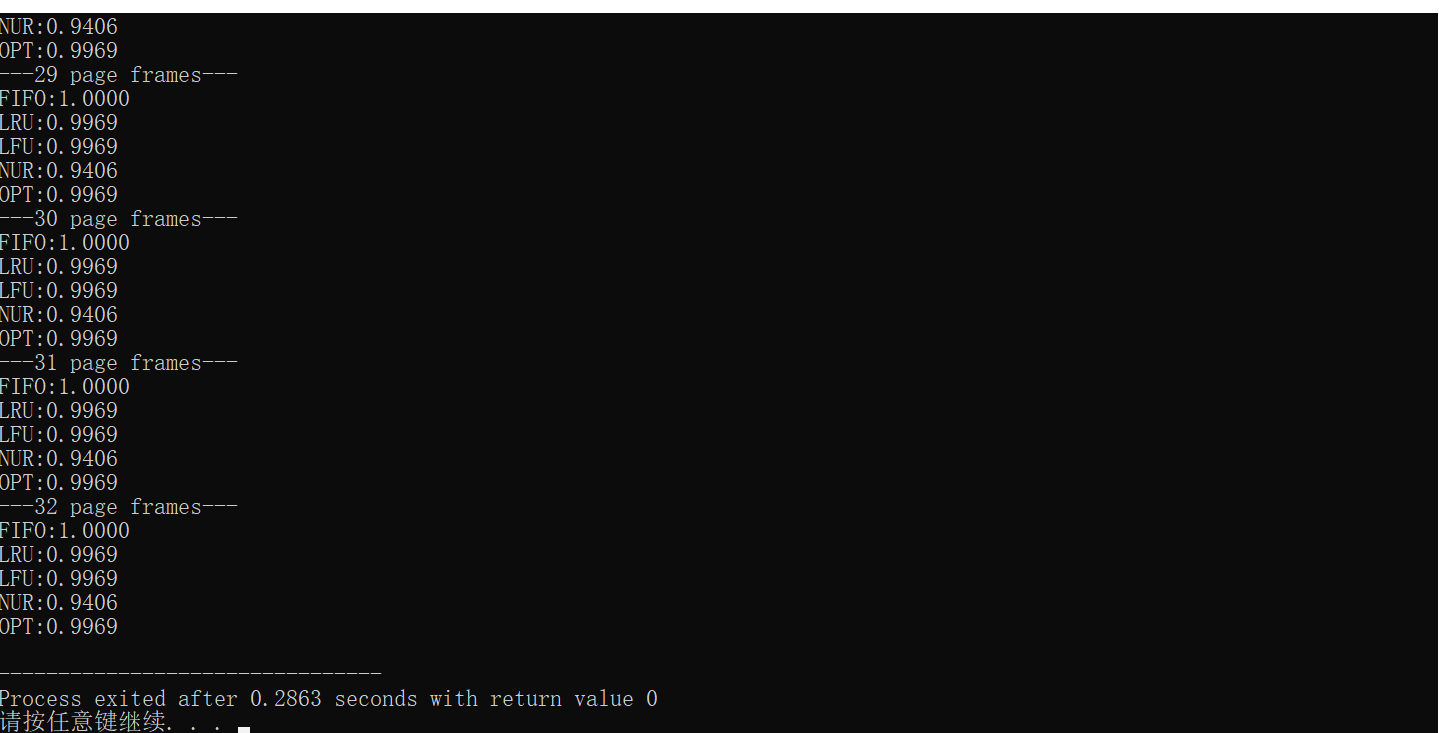展开全文• 实验四 页式虚拟存储管理中地址转换和页式中断 FIFO 一实验目的 深入了解页式存储管理如何实现地址转换进一步认识页式虚拟存储管理中如何处理缺页中断以及页面置换算法 二实验主要内容 编写程序完成页式虚拟存储管理...
•页面置换算法
• 操作系统 页面置换算法FIFOLRU

# FIFO

FIFO算法是最早出现的置换算法。该算法总是淘汰最先进入内存的页面，即选择在内存中驻留时间最久的页面予以淘汰。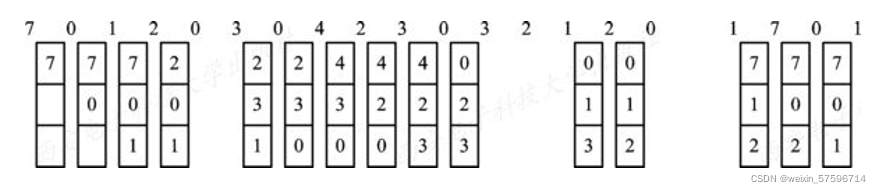# LRU

最近最久未使用(LRU)的页面置换算法是根据页面调入内存后的使用情况做出决策的，需要记录页面的访问时间。每当进程访问某页面时， 便将该页面的页面号从栈中移出，将它压入栈顶。因此，栈顶始终是最新被访问页面的编号，而栈底则是最近最久未使用页面的页面号。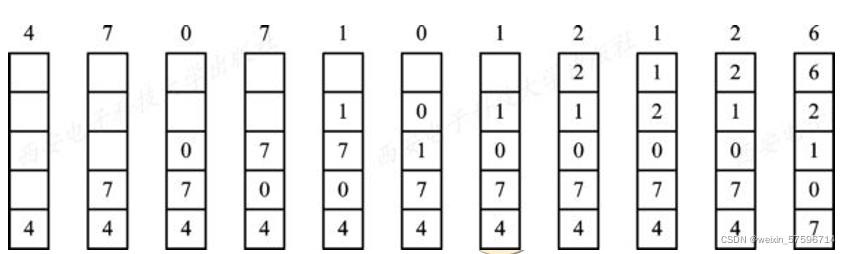# 代码实现

#include<conio.h>
#include<stdio.h>
#include<stdlib.h>
#include<string.h>
#define Myprintf printf("|---+---+---+---+---+---+---+---+---+---+---+---+---+---+---+---+---|\n") //表格控制
#define bsize 4     //物理块大小
#define psize 16     //进程大小
typedef struct page{
int num;  //记录页面号
int time;  //记录调入内存时间
}Page;                   //页面逻辑结构，结构为方便算法实现设计

Page b[bsize];            //内存单元数
int c[bsize][psize];   //暂保存内存当前的状态：缓冲区
int queue;      //记录调入队列
int K;              //调入队列计数变量
int phb[bsize] = {0};   //物理块标号
int pro[psize] = {0};     //进程序列号
int flag[bsize] = {0};  //进程等待次数(存放最久未被使用的进程标志)
int i = 0, j = 0, k = 0;   //i表示进程序列号,j表示物理块号
int m = -1, n = -1;       //物理块空闲和进程是否相同判断标志
int max = -1,maxflag = 0; //标记替换物理块进程下标
int count = 0;            //统计页面缺页次数

int* build(){
//随机产生序列号函数
printf("随机产生一个进程序列号为：\n");
int i = 0;
for(i=0; i<psize; i++){
pro[i] = 10*rand()/(RAND_MAX+1)+1;
printf("%d  ",pro[i]);
}
printf("\n");
return(pro);
}

int searchpb(){
//查找空闲物理块
for(j=0; j<bsize; j++){
if(phb[j] == 0){
m = j;
return m;
break;
}
}
return -1;
}

int searchpro(){
//查找相同进程
for(j = 0; j < bsize; j++){
if(phb[j] == pro[i]){
n = j;
return j;
}
}
return -1;
}

void empty(){
//初始化内存
for(i=0;i<bsize;i++){
phb[i]=0;
}
count=0;                //计数器置零
}

void FIFO(){
//先进先出页面置换算法
for(i = 0; i<psize; i++){
m=searchpb();
n=searchpro();
for(j = 0; j < bsize;j++){
//找flag值最大的
if(flag[j]>maxflag){
maxflag = flag[j];
max = j;
}
}
if(n == -1){
//不存在相同进程
if(m != -1){
//存在空闲物理块
phb[m] = pro[i];   //进程号填入该空闲物理块
count++;
flag[m] = 0;
for(j = 0;j <= m; j++){
flag[j]++;
}
m = -1;
}
else{
//不存在空闲物理块
phb[max] = pro[i];
flag[max] = 0;
for(j = 0;j < bsize; j++){
flag[j]++;
}
max = -1;
maxflag = 0;
count++;
}
}
else{
//存在相同的进程
phb[n] = pro[i];
for(j = 0;j < bsize; j++){
flag[j]++;
}
n = -1;
}
for(j = 0 ;j < bsize; j++){
printf("%d  ",phb[j]);
}
printf("\n");
}
printf("缺页次数为：%d\n",count);
printf("\n");
}

void Init(Page *b,int c[bsize][psize]){
//初始化内存单元、缓冲区
int i,j;
for(i=0;i<psize;i++){
b[i].num=-1;
b[i].time=psize-i-1;
}
for(i=0;i<bsize;i++)
for(j=0;j<psize;j++)
c[i][j]=-1;
}

int GetMax(Page *b){
//取得在内存中停留最久的页面,默认状态下为最早调入的页面
int i;
int max=-1;
int tag=0;
for(i=0;i<bsize;i++){
if(b[i].time>max){
max=b[i].time;
tag=i;
}
}
return tag;
}

int Equation(int fold,Page *b){
//判断页面是否已在内存中
int i;
for(i=0;i<bsize;i++){
if (fold==b[i].num)
return i;
}
return -1;
}

void Lruu(int fold,Page *b){
//LRU核心部分
int i;
int val;
val=Equation(fold,b);
if (val>=0){
b[val].time=0;
for(i=0;i<bsize;i++)
if (i!=val)
b[i].time++;
}
else{
queue[++K]=fold; //记录调入页面
val=GetMax(b);
b[val].num=fold;
b[val].time=0;
for(i=0;i<bsize;i++)
if (i!=val)
b[i].time++;
}
}

void LRU(){
int i,j;
K=-1;
Init(b, c);
for(i=0;i<psize;i++){
Lruu(pro[i],b);
c[i]=pro[i];
//记录当前的内存单元中的页面
for(j=0;j<bsize;j++)
c[j][i]=b[j].num;
}
//结果输出
printf("内存状态为：\n");
Myprintf;
for(j=0;j<psize;j++)
printf("|%2d ",pro[j]);
printf("|\n");
Myprintf;
for(i=0;i<bsize;i++){
for(j=0;j<psize;j++){
if(c[i][j]==-1)
printf("|%2c ",32);
else
printf("|%2d ",c[i][j]);
}
printf("|\n");
}
Myprintf;
printf("\n调入队列为:");
for(i=0;i<K+1;i++)
printf("%3d",queue[i]);
printf("\n缺页次数为：%6d\n缺页率：%16.6f",K+1,(float)(K+1)/psize);
}

int main()
{
int sel;
do{
printf("0、退出(Exit)\n");
printf("1、产生随机序列\n");
printf("2、最久未使用(LRU)\n");
printf("3、先进先出(FIFO)\n");
printf("<请选择所要执行的操作:(0)(1)(2)(3)>\n");
scanf("%d",&sel);
if(sel == 0){
printf("退出 \n");
}
if(sel == 1){
build();
}
if(sel == 2){
printf("LRU\n");
LRU();
empty();
printf("\n");
}
if(sel == 3){
printf("FIFO\n");
FIFO();
empty();
printf("\n");
}
}while(sel!=0);
}


# 效果图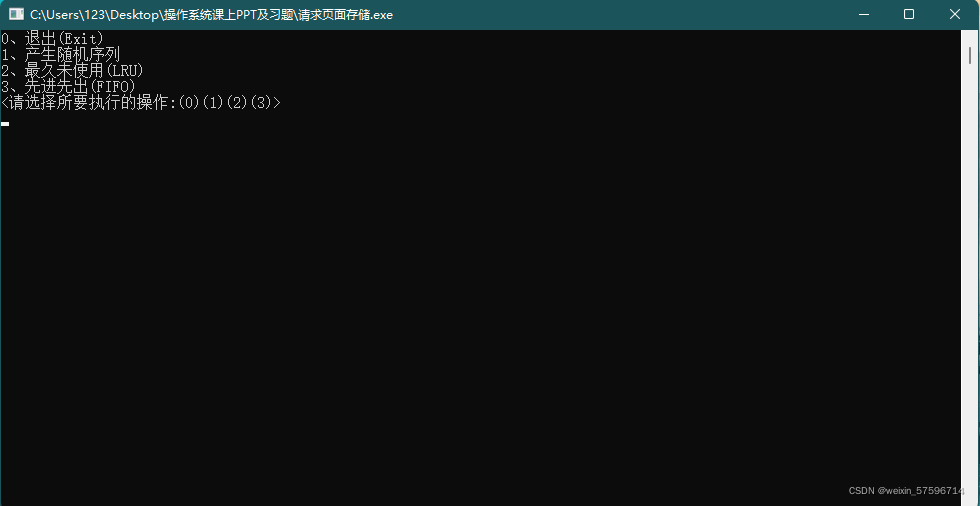先输入1，产生随机序列，模拟进程序号列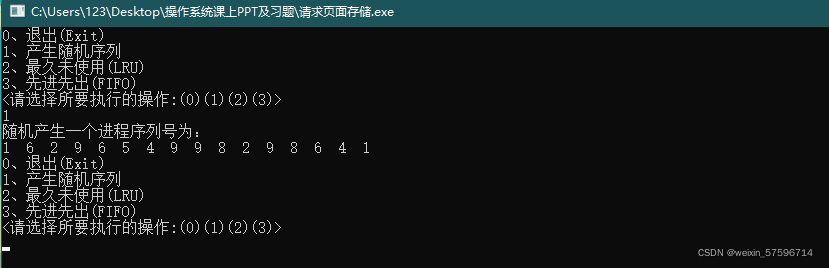再输入2调用LRU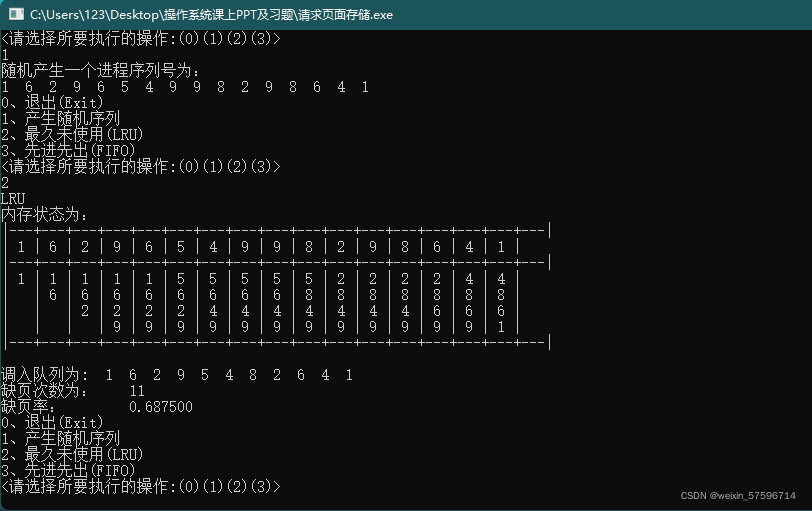输入3调用FIFO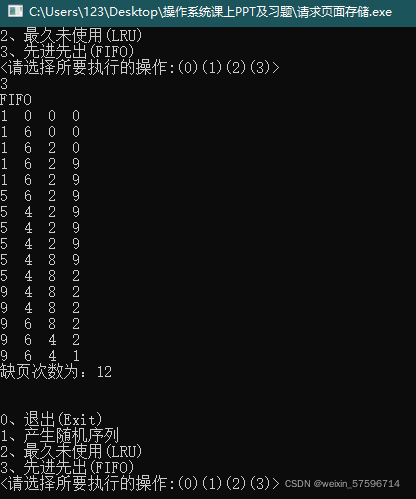输入0退出

展开全文操作系统 页面置换算法
• 1 FIFO算法（先进先出） 2 2 最近最久未使用算法（LRU算法）基本思想 2 二 程序设计 2 1 数据结构设计 2 2 函数设计 3 3 流程图 5 1 FIFO算法设计流程图 5 2 LRU 算法设计流程图： 6 三 代码 8 四 结果分析 12 五 ...
• 随机给出一个页面执行序列，如：1,5,3,4,2,1,3,4,5,7,9,……。要求计算以下几种置换算法的缺页数、缺页率和命中率。 最佳置换算法OPT（Optimal） ...最近最少使用算法LRU（Least Recently Used）
• 自己写的 希望有错提出来 期中那个ＯＰＴ写的不是很好，希望可以多多指教
• 该工程具体是在codeblock上面实现了操作系统课程上讲解的页面置换算法，包括先进先出（FIFO）、最佳置换算法（OPT）、最久最近未使用算法（LRU）。 具体实现功能有： 1、建立相应的数据结构 2、在屏幕上显示页面的...页面置换算法
• 缓存算法（FIFOLRU、LFU三种算法的区别） FIFO算法# FIFO 算法是一种比较容易实现的算法。它的思想是先进先出（FIFO，队列），这是最简单、最公平的一种思想，即如果一个数据是最先进入的，那么可以认为在将来...
• C语言 页面置换算法 OPT FIFO LRU clock
• FIFO+LRU算法——图文讲解 例1：在一个采用页式虚拟存储管理的系统中，有一用户作业，它依次要访问的页面序列是1，2，3，4，1，2，5，1，2，3，4，5.假定分配给该作业的页数为3且作业初始时未装载页面，那么采用FIFO...经验分享
• 如何编程思想FIFOLRU算法，写一个程序来实现本章中介绍的FIFOLRU页置换算法。首先产生一个随机的页面引用序列，页面数从0~9。将这个序列应用到每个算法并记录发生的页错误的次数。实现这个算法时，要将页帧的...操作系统
• FIFO：First In First Out，先进先出LRU：Least Recently Used，最近最少使用 LFU：Least Frequently Used，最不经常使用 以上三者都是缓存过期策略。 原理和实现： 一、FIFO按照“先进先出（FirstIn，FirstOut...
• 该工程具体是在codeblock上面实现了操作系统课程上讲解的页面置换算法，包括先进先出（FIFO）、最佳置换算法（OPT）、最久最近未使用算法（LRU）。 具体实现功能有： 1、建立相应的数据结构 2、在屏幕上显示页面...
• c语言 操作系统 请求分页 OPT FIFO LRU算法 源码
• 操作系统之存储管理——FIFO算法和LRU算法 操作系统之磁盘调度——SCAN实例讲解 一、实验目的 存储管理的主要功能之一是合理地分配空间。请求页式管理是一种常用的虚拟存储管理技术。 本实验的目的是通过请求...操作系统
• 操作系统 OPT FIFO LRU 算法，有详细注释
• FIFO：先进先出调度算法 最先加载到内存的最先被置换出去 LRU：最近最久未使用调度算法 最近最少被访问的最先被置换出去 两者都是缓存调度算法，经常用作内存的页面置换算法。 打一个比方，帮助你理解。 你有很多的......